# Right, Straight, and Complete Angle by Direction and Clock

#### definition

Right Angle: The right angle is exactly equal to 90°.

Straight Angle: A straight angle is equal to 180°.

Revolution: Turning by two straight angles (or four right angles) in the same direction makes a full turn. This one complete turn is called one revolution.

Complete Angle: The angle for one revolution is a complete angle. A complete angle is one complete revolution or equal to 360°.

# Right, Straight, and Complete Angle by Direction and Clock:

Let us understand the concept of right and straight angles by directions.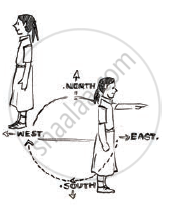When we move from North to East then it forms an angle of 90° which is called Right Angle.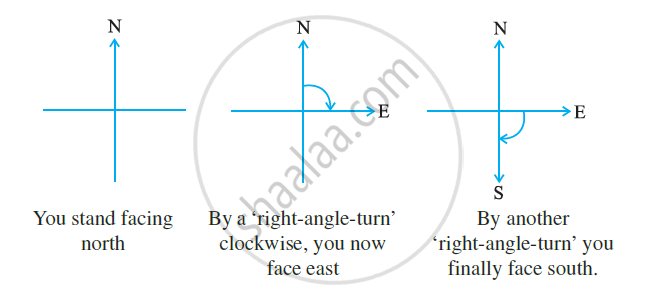When we move from North to South then it forms an angle of 180° which is called Straight Angle.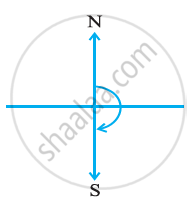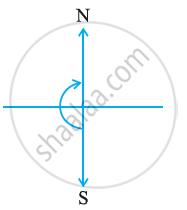When we move four right angles in the same direction then we reach to the same position again i.e. if we make a clockwise turn from North to reach to North again then it forms an angle of 360° which is called a Complete Angle. This is called one revolution.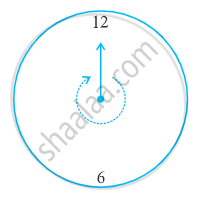In a clock, there are two hands i.e. minute hand and hour hand, which moves clockwise in every minute. When the clock hand moves from one position to another then turns through an angle.

When a hand starts from 12 and reaches to 12 again then it is said to be completed a revolution.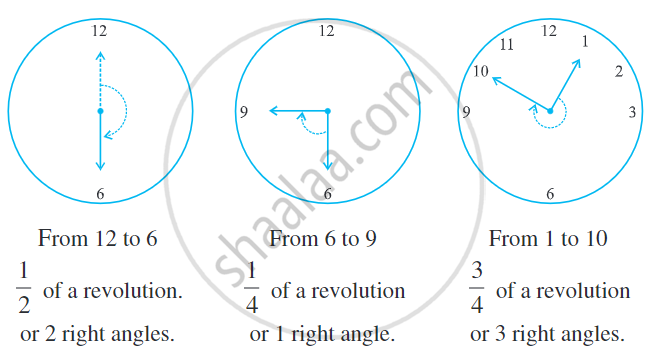If you would like to contribute notes or other learning material, please submit them using the button below.

### Shaalaa.com

Right Angle, Straight Angle, Complete Angle & Reflex Angle Part-1 [00:16:42]
S